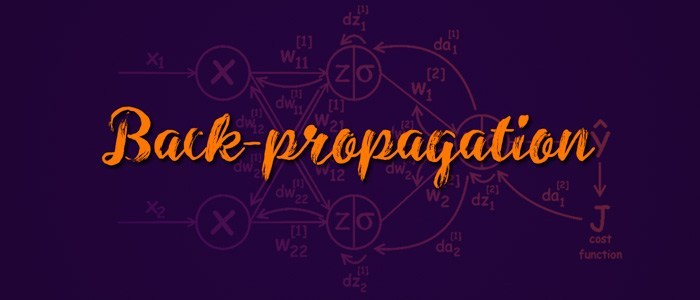/  Technology   /  Back Propagation and Computational Graphs in Neural Networks## Back Propagation:

In Deep Learning , you are definitely heard about the back propagation at least once. In this article I am going to explain about the Back propagation.

The entire process of deep learning training may be summarized into following steps.

1. Data Analysis and Data Exploration.
2. Based on our Data Analysis information we have to build a framework such as TensorFlow , PyTorch etc.
3. Now choose the best appropriate cost function like mean squared error or binary cross entropy depends on our data.
4. After selecting the cost function we have to select the optimizer i.e Gradient Descent , Stochastic gradient Descent , Adam etc..
5. Now, train our model to minimize or reduce the selected loss function w.r.to network parameters using the training examples.
6. And finally , analyzing the performance of the network.

Generally , in neural networks the forward feed propagation refers to flow of the data which maps input to the output of the network. After forward propagation for an input p  to get an output q . using the output value(Predicted value) . the cost function is computed for the training examples.

The back propagation algorithm is allows the calculation of the gradients for the optimization techniques. People thinks that the back propagation is learning algorithm foe whole neural network. But it is not a learning algorithm it is just a technique for the calculation of gradients during its optimization stage.The back-propagation algorithm holds the chain rule of differential equations to compute the error gradients In summation of local-gradient products over the various paths from a node to output. The back-propagation is a direct application of dynamic programming.

## Computational Graphs:

The computational graphs are a method to representing the mathematical expressions. In this we are having a two types of computations.

1. Forward Computation.

2. Backward computations.

In computational graphs few terms are need to know.

Node:

The node indicates a variable which may be scalar , vector , tensor or another type of variable.

Edge:

The edge represents a function arguments and data dependency. These are just like pointers to nodes.

Operation:

These operations are simple functions of one or  more variables. In this a fixed set of operations are there.

A node is used to compute the its value and the value of its derivative based on each argument (Edge) times a derivative of an arbitrary input.

In simple words the nodes represents the units of computation and edges represents the data produced or consumed by a computation. the most important advantage of computation is the edges represents the dependencies between the operations. It will makes the identification of operations that can be executed in parallel easier. The deep learning frameworks are PyTorch , TensorFlow also depends on the creation of these computational graphs to implement the back propagation for defined networks. The example of computational graphs are shown below.This graph is used to compute the values of the expressions using the bottom up data flow.

For example:Chain Rule of Calculus:

This chain rule is the one type of derivative method for computing the derivative of two or more functions. This may be written in Leibniz’s notation. If a variable z depends on the variable y which itself depends on the variable x , then y and z are dependent variables and z is the intermediate variable of y depends on x as well. Then the chain rule states thatThe below figure shows the chain ruleThe partial derivative of the any node a with respect to any node b in the above graph is equal to the aggregated sum of product of partial derivatives of edges of all the paths from n ode b to a. this will shown in above figure where there is two paths from the input node to output node which includes in derivative calculation.

Back-propagation applied to our example graph is shown below. Starting from the output node partial derivatives are calculated for all the lower nodes.Once the backward graph is built, calculating derivatives is straightforward and it is heavily optimized in the deep learning frameworks.

To summarize, in neural networks to calculate the gradients, the path-aggregation is over exponentially increasing number of paths, which seems to be intractable at first sight. However, back-propagation allows this calculation in an efficient manner (a type of dynamic programming technique).